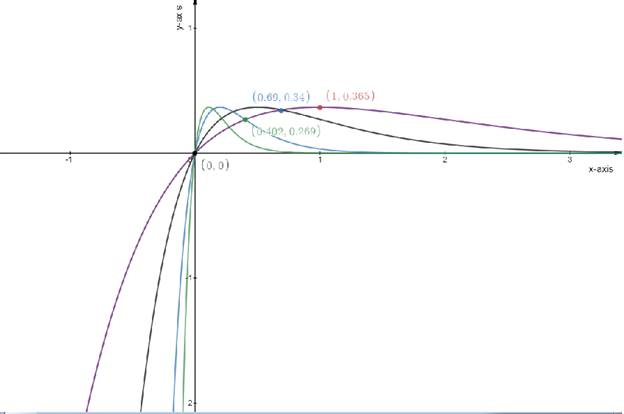# The features of the family members of the curve.### Single Variable Calculus: Concepts...

4th Edition
James Stewart
Publisher: Cengage Learning
ISBN: 9781337687805### Single Variable Calculus: Concepts...

4th Edition
James Stewart
Publisher: Cengage Learning
ISBN: 9781337687805

#### Solutions

Chapter 4, Problem 24RE
To determine

## The features of the family members of the curve.

Expert Solution

### Explanation of Solution

Given:

The function is

f(x)=cxecx

Concept used:

The slope of the tangent to a curve f(x) at any point a is given by the value of the first derivative of the slope at the point x=a .

The tangent to be horizontal so the slope should be equal to 0

That is dydx=0 .

Calculation:

The function is

f(x)=cxecx................................(1)

Using the value c=1,2,5and9

y=xexy=2xe2xy=5xe5xy=9xe9x

Draw the table

f(x)=cxecx

Test one point in each of the region formed by the graph

If the point satisfies the function then shade the entire region to denote that every point in the region satisfies the function

 x−axis 0 1 0.69 0.402 y−axis 0 0.36 0.34 0.269

Draw the graph

f(x)=cxecx### Have a homework question?

Subscribe to bartleby learn! Ask subject matter experts 30 homework questions each month. Plus, you’ll have access to millions of step-by-step textbook answers!